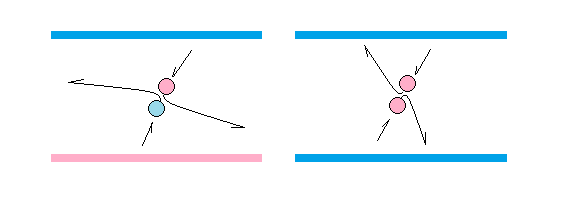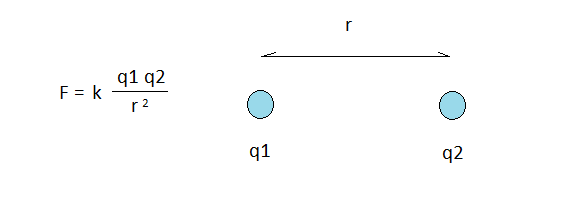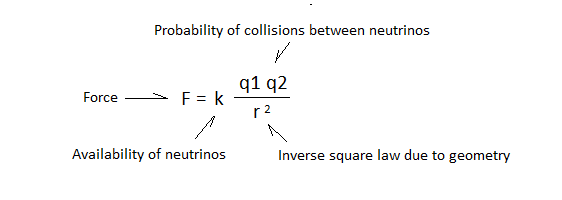skip to Main Content

To understand the electric force, gravity and magnetism, we must return to our definition of the aether, because it is the aether that makes action at a distance possible.

We have to keep in mind that the aether is so dense that every particle in it is in physical contact with every neighbouring particle. This means that if we can manipulate the aether between two surfaces in such a way that some of its particles leave this field, we get tension, forcing the surfaces together. Conversely, if we can manipulate the aether in such a way that particles get sucked into this field, there will be pressure, forcing the surfaces apart. Unless we re-establish equilibrium, there will be tension or pressure, depending on the situation.

Let us further consider what we have said about textures of particles, and the fact that neutrinos are of mixed texture. Neutrinos receive footprints of whatever surface they were last in contact with. This is information that neutrinos take with them as they return back into the field.

Now, consider what happens when a neutrino with a woolly footprint comes in contact with a neutrino with an abrasive footprint. There is a degree of affinity between the two neutrinos. They latch on to each other. On the other hand, if two neutrinos of identical texture collide, there is virtually no affinity. This means that collisions between equally charged neutrinos are different from collisions of differently charged neutrinos. In fact, we can make the following claim based on observation:

Neutrinos of opposite charge collide in such a way that they have a tendency to leave the field, while neutrinos of identical charge collide in such a way that they have a tendency to stay in the field.Collision of differently charged neutrinos compared to collision of equally charged neutrinos

With this model, we have an explanation for why surfaces of opposite charge attract each other, while surfaces of same charge repel each other. It all boils down to the neutrinos in the aether and how they tend to leave the field when differently charged, and stay in the field if equally charged.

A consequence of this is that there must be electric pressure inside electrons and protons. The walls inside electrons are predominantly negatively charged, and the walls inside protons are predominantly positively charged. In both cases we have a situation in which neutrinos will tend to stay inside. This makes electrons and protons more like inflated balls than saggy balloons. It makes them bouncy, as required for the bouncing electron hypothesis.

On a final note, the relationship between the aether and what we call space should not be forgotten. Space is a void filled with aether. When we manipulate the aether, we are in fact manipulating space itself.

## Coulomb’s law as availability, probability and geometry

Let us now consider Coulomb’s law to see if we can arrive at this ourselves by simply applying what we have discussed so far.Coulomb’s Law

Coulomb’s law states that the force of attraction or repulsion between two point charges, q1 and q2, can be calculated from the strength of the charges themselves, the distance separating them, r, and a constant k. The formula states that the force F equals k multiplied by the product of q1 and q2, divided by the square of r.

This can be related to our theory as follows:

1. Let k represent the general availability of neutrinos in the aether.
2. Let q1 and q2 represent the probability of collisions between charged neutrinos.
3. Let r represent the diminishing chance of collisions with distance.

While point 1 requires no further explanation, we need to explain point 2 and 3. It is not immediately clear why q1 should be multiplied by q2, nor why r should be squared.

When it comes to q1 and q2, we have to keep in mind that footprints left on neutrinos are directly related to the charge on the point charges. Q1 and q2 are therefore proxy values for how full the aether is of charged neutrinos.

Furthermore, we have to recognize that collisions are probabilistic events. Such events are calculated using multiplication. When we are talking about very large numbers of collisions, the way we calculate the grand total is also by multiplication. Q1 must therefore be multiplied by q2 in order to give us a value reflecting the overall total of collisions.

When it comes to the distance r, we have to recognize that charged neutrinos are more densely distributed close to the point charges, and that this distribution tapers off by the square of the distance. This is the inverse square law, which can be derived directly from geometry.

From this, we can now calculate the overall number of neutrino collisions by multiplying k with the product of q1 and q2, divided by the square of r. Keeping in mind that it is neutrino collisions that produce force by pumping aether into or out of the field between charges, we have arrived at Coulomb’s law:Coulomb’s Law Explained

From this it is clear that it is possible to see Coulomb’s law as an expression related to the aether and the probability of collisions happening in it.

We can also conclude that Coulomb’s law must break down at extremely short distances, such as those found inside atoms. This is because this law relates to collisions in the aether. When the distance between two point charges goes to zero, the number of neutrinos between them go to zero as well.

This explains why electrons are only loosely attracted to atomic nuclei when they are in physical contact. With no aether to provide an electric force between the two particles, there’s only their respective textures that keep them together.

For Coulomb’s law to work according to formula, charges must be separated by a minimum distance, and it is this fact that allows an electron at rest on an atomic nucleus to move sufficiently high to start bouncing. The electric force at the surface of a charged particle is not infinite or close to infinite, it’s zero. Very close to the surface it’s near zero. Then the force quickly peeks before tapering off with distance according to the inverse square law. Only then does things behave fully according to Coulomb’s law.

In a pure particle model where everything, including space comes in discrete quanta, conventional formulas tend to break down at extremely small scales. This is because most formulas model reality as a continuous whole, while pure particle models see reality as something composed of discrete quanta.

< Electron orbits ———— | ———— Gravity >

Back To Top

By continuing to use the site, you agree to the use of cookies. More information

The cookie settings on this website are set to "allow cookies" to give you the best browsing experience possible. If you continue to use this website without changing your cookie settings or you click "Accept" below then you are consenting to this.

Close